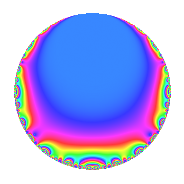# Properties

 Label 162.2.a.cLevel 162 Weight 2 Character orbit 162.a Self dual yes Analytic conductor 1.294 Analytic rank 0 Dimension 1 CM no Inner twists 1

# Related objects

## Newspace parameters

 Level: $$N$$ = $$162 = 2 \cdot 3^{4}$$ Weight: $$k$$ = $$2$$ Character orbit: $$[\chi]$$ = 162.a (trivial)

## Newform invariants

 Self dual: yes Analytic conductor: $$1.29357651274$$ Analytic rank: $$0$$ Dimension: $$1$$ Coefficient field: $$\mathbb{Q}$$ Coefficient ring: $$\mathbb{Z}$$ Coefficient ring index: $$1$$ Twist minimal: no (minimal twist has level 18) Fricke sign: $$-1$$ Sato-Tate group: $\mathrm{SU}(2)$

## $q$-expansion

 $$f(q)$$ $$=$$ $$q + q^{2} + q^{4} + 2q^{7} + q^{8} + O(q^{10})$$ $$q + q^{2} + q^{4} + 2q^{7} + q^{8} - 3q^{11} + 2q^{13} + 2q^{14} + q^{16} - 3q^{17} - q^{19} - 3q^{22} - 6q^{23} - 5q^{25} + 2q^{26} + 2q^{28} + 6q^{29} - 4q^{31} + q^{32} - 3q^{34} - 4q^{37} - q^{38} + 9q^{41} - q^{43} - 3q^{44} - 6q^{46} - 6q^{47} - 3q^{49} - 5q^{50} + 2q^{52} + 12q^{53} + 2q^{56} + 6q^{58} + 3q^{59} + 8q^{61} - 4q^{62} + q^{64} + 5q^{67} - 3q^{68} - 12q^{71} + 11q^{73} - 4q^{74} - q^{76} - 6q^{77} - 4q^{79} + 9q^{82} + 12q^{83} - q^{86} - 3q^{88} + 6q^{89} + 4q^{91} - 6q^{92} - 6q^{94} + 5q^{97} - 3q^{98} + O(q^{100})$$

## Embeddings

For each embedding $$\iota_m$$ of the coefficient field, the values $$\iota_m(a_n)$$ are shown below.

For more information on an embedded modular form you can click on its label.

Label $$\iota_m(\nu)$$ $$a_{2}$$ $$a_{3}$$ $$a_{4}$$ $$a_{5}$$ $$a_{6}$$ $$a_{7}$$ $$a_{8}$$ $$a_{9}$$ $$a_{10}$$
1.1
 0
1.00000 0 1.00000 0 0 2.00000 1.00000 0 0
 $$n$$: e.g. 2-40 or 990-1000 Significant digits: Format: Complex embeddings Normalized embeddings Satake parameters Satake angles

## Inner twists

This newform does not admit any (nontrivial) inner twists.

## Twists

By twisting character orbit
Char Parity Ord Mult Type Twist Min Dim
1.a even 1 1 trivial 162.2.a.c 1
3.b odd 2 1 162.2.a.b 1
4.b odd 2 1 1296.2.a.g 1
5.b even 2 1 4050.2.a.c 1
5.c odd 4 2 4050.2.c.c 2
7.b odd 2 1 7938.2.a.x 1
8.b even 2 1 5184.2.a.r 1
8.d odd 2 1 5184.2.a.o 1
9.c even 3 2 18.2.c.a 2
9.d odd 6 2 54.2.c.a 2
12.b even 2 1 1296.2.a.f 1
15.d odd 2 1 4050.2.a.v 1
15.e even 4 2 4050.2.c.r 2
21.c even 2 1 7938.2.a.i 1
24.f even 2 1 5184.2.a.p 1
24.h odd 2 1 5184.2.a.q 1
36.f odd 6 2 144.2.i.c 2
36.h even 6 2 432.2.i.b 2
45.h odd 6 2 1350.2.e.c 2
45.j even 6 2 450.2.e.i 2
45.k odd 12 4 450.2.j.e 4
45.l even 12 4 1350.2.j.a 4
63.g even 3 2 882.2.h.c 2
63.h even 3 2 882.2.e.i 2
63.i even 6 2 2646.2.e.c 2
63.j odd 6 2 2646.2.e.b 2
63.k odd 6 2 882.2.h.b 2
63.l odd 6 2 882.2.f.d 2
63.n odd 6 2 2646.2.h.h 2
63.o even 6 2 2646.2.f.g 2
63.s even 6 2 2646.2.h.i 2
63.t odd 6 2 882.2.e.g 2
72.j odd 6 2 1728.2.i.e 2
72.l even 6 2 1728.2.i.f 2
72.n even 6 2 576.2.i.g 2
72.p odd 6 2 576.2.i.a 2

By twisted newform orbit
Twist Min Dim Char Parity Ord Mult Type
18.2.c.a 2 9.c even 3 2
54.2.c.a 2 9.d odd 6 2
144.2.i.c 2 36.f odd 6 2
162.2.a.b 1 3.b odd 2 1
162.2.a.c 1 1.a even 1 1 trivial
432.2.i.b 2 36.h even 6 2
450.2.e.i 2 45.j even 6 2
450.2.j.e 4 45.k odd 12 4
576.2.i.a 2 72.p odd 6 2
576.2.i.g 2 72.n even 6 2
882.2.e.g 2 63.t odd 6 2
882.2.e.i 2 63.h even 3 2
882.2.f.d 2 63.l odd 6 2
882.2.h.b 2 63.k odd 6 2
882.2.h.c 2 63.g even 3 2
1296.2.a.f 1 12.b even 2 1
1296.2.a.g 1 4.b odd 2 1
1350.2.e.c 2 45.h odd 6 2
1350.2.j.a 4 45.l even 12 4
1728.2.i.e 2 72.j odd 6 2
1728.2.i.f 2 72.l even 6 2
2646.2.e.b 2 63.j odd 6 2
2646.2.e.c 2 63.i even 6 2
2646.2.f.g 2 63.o even 6 2
2646.2.h.h 2 63.n odd 6 2
2646.2.h.i 2 63.s even 6 2
4050.2.a.c 1 5.b even 2 1
4050.2.a.v 1 15.d odd 2 1
4050.2.c.c 2 5.c odd 4 2
4050.2.c.r 2 15.e even 4 2
5184.2.a.o 1 8.d odd 2 1
5184.2.a.p 1 24.f even 2 1
5184.2.a.q 1 24.h odd 2 1
5184.2.a.r 1 8.b even 2 1
7938.2.a.i 1 21.c even 2 1
7938.2.a.x 1 7.b odd 2 1

## Atkin-Lehner signs

$$p$$ Sign
$$2$$ $$-1$$
$$3$$ $$1$$

## Hecke kernels

This newform subspace can be constructed as the intersection of the kernels of the following linear operators acting on $$S_{2}^{\mathrm{new}}(\Gamma_0(162))$$:

 $$T_{5}$$ $$T_{11} + 3$$